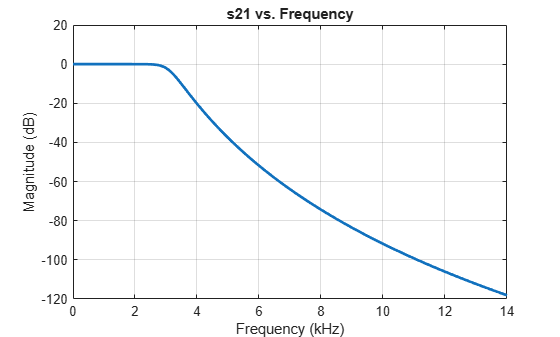# setrfplot

Set axis type for `rfplot` in RF Toolbox

## Syntax

``setrfplot(axistype)``
``setrfplot(axistype,persist)``

## Description

example

````setrfplot(axistype)` applies or removes the use of engineering units on the X-axis of `rfplot`. By default, engineering units are always applied in the X-axis and persist across all MATLAB sessions.```
````setrfplot(axistype,persist)` controls the persistence behavior of the units on the X-axis plot across MATLAB sessions.```

## Examples

collapse all

This example shows how to design a low-pass Butterworth filter with passband frequency of 3 kHz, stopband frequency 7 kHz, passband attenuation of 2 dB, and stopband attenuation 60 dB. Display the filter order of such a designed filter and determine the passband frequency at 3.0103 dB. See  in `rffilter` object page.

Filter Parameters

```Fp = 3e3; % Passband frequency, Hz Ap = 2; % Passband attenuation, dB Fs = 7e3; % Stopband frequency, Hz As = 60; % Stopband attenuation, dB```

Design Filter

```r = rffilter("FilterType","Butterworth","ResponseType","Lowpass","Implementation","Transfer function","PassbandFrequency",Fp, ... "PassbandAttenuation",Ap,"StopbandFrequency",Fs,"StopbandAttenuation",As);```

Filter Order of Designed Filter

```N = r.DesignData.FilterOrder; sprintf('Calculated filter order is %d',N)```
```ans = 'Calculated filter order is 9' ```

Frequency at 3.0103 dB

```F_3dB = r.DesignData.PassbandFrequency/1e3; sprintf('Frequency at 3.0103 dB is %d kHz',F_3dB)```
```ans = 'Frequency at 3.0103 dB is 3.090733e+00 kHz' ```

Visualize Magnitude Response

```frequencies = linspace(0,2*Fs,1001); rfplot(r, frequencies)```Note: To use `rfplot` and `plot` on the same figure use `setrfplot`. Type `'help setrfplot`' in command window for information.

Reference

1. Larry D. Paarmann, Design and Analysis of Analog Filters: A Signal Processing Perspective, Kluwer Academic Publishers

## Input Arguments

collapse all

Units on `rfplot` X-axis, specified as `'engunits'` or `'noengunits'`.

Data Types: `char` | `string`

Units on `rfplot` X-axis across MATLAB sessions, specified as `true` or `false`.

Data Types: `logical`

## Version History

Introduced in R2019b